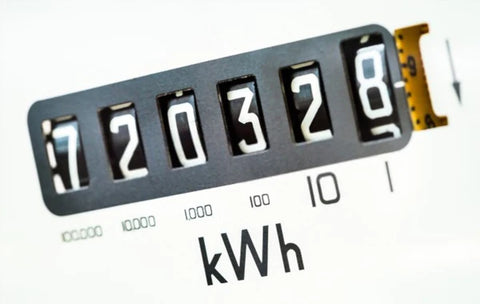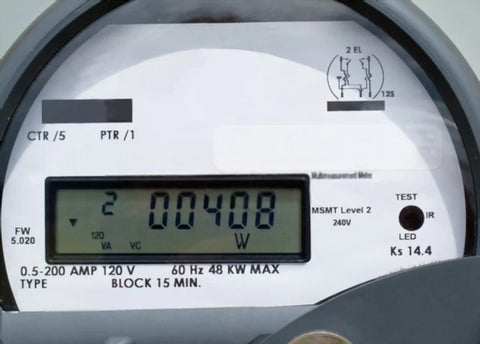Amp Hour Calculator (Battery Capacity Calculator) - ShopSolar.com

# Amp Hour Calculator (Battery Capacity Calculator)

When it comes to understanding battery capacity, amp hours (Ah) are one of the most important things to know about.

An amp hour is the amount of energy that 1 amp can discharge in 1 hour. It is used when talking about energy storage, hence why it is vital when dealing with batteries.

This applies to the batteries you use to power your electronics but becomes even more relevant when we are talking about large batteries or seep-cycle batteries.

Error: This field is required.
Error: This field is required.
Optional: If you select a battery type, we ’ll tell you how much usable capacity your battery bank has.
How many batteries do you have in your battery bank? If you have more than 1, we ’ll ask how they ’re wired together.
Error: This field is required.

Battery voltage:

## What Are Amp Hours?Firstly, you need to fully understand what an amp is. It’s a shortened form of the official name for the unit, which is ‘amperes’.

Amps measure the electrical current and tell us how fast it is going. It’s represented by ‘I’ in scientific and mathematical formulas.

Amp-hours, on the other hand, is a more directly useful way to understand your battery. It tells you how long your battery will last. Amp hours are most commonly used to understand the capacity and lifespan of a battery.

When it comes to the relationship between amps and amp hours, it is about how we use them practically. The Ah of a battery tells us how long it will take for the electrical current of the battery to fully discharge.

It’s important that we always use hours when dealing with battery capacity, as that is how the formulas are designed.

## Amp-Hours Calculator

Firstly, you need to understand how to calculate amp hours. In order to calculate amp hours, you need to know some other information about your battery.

The first thing you need to know is the voltage of your battery. This should be clearly indicated on the battery itself. Volts are units of electric potential.

In most cases, you will not need to calculate the voltage, but there is also an amps-to-volts calculator should you need to actually work it out.

The other piece of information that you need to know is the watt hours of your battery. Luckily, most devices show their wattage clearly.

To convert watts to watt-hours (Wh), you take the wattage (W) and multiply it by the hours (h) it is in use. As a simplified formula, this would be written as Wh = W x h. It is important to always use time in hours.Once you know the watt hours and the voltage, you can calculate the Ah. There are a few formulas that you can use to work it out - we’ll explain the process and then give you the one you need to actually calculate amp hours.

### Formulas

Firstly, Power (P) is equal to Voltage (V) x Current (I). Power is the rate at which current moves, and is measured in watts. We have already covered voltage and current, which are measured in volts and amps respectively.

Energy refers to what is delivered by the circuit, and it is calculated by multiplying Power by Time (T) in hours. If we multiply both sides of the previous formula by time on both sides of the equation, we get the following equation:

E = V x I x T.

The “I x T” part of this equation is the current in amps multiplied by time in hours. This then gives us the amp hours of the battery. You can use this formula to calculate whichever variables you don’t have.

### Battery Amp-Hour Calculator

But this formula is a bit complicated, and there is an easier way to work out the Ah of your battery.

To work out the amp hours, you simply need to divide the watt-hours by the voltage. That looks like Ah = Wh/V.

For example, the Bluetti AC200 max has 2,048Wh, and a voltage of 51.2 V. To work out the Ah, you would take 2,048 / 51.2 and end up with 40 Ah.

This means that your Bluetti can provide 40 A for 1 hour, or 20 A for 2 hours, and so on.

### Battery Ah Rating Calculator

When it comes to comparing the Ah rating of different batteries, ones with higher Ah ratings will last longer. This is because they hold more charge.

The Ah rating of a battery is just another way of describing the number of amps that a battery can produce in 1 hour. A 20 Ah battery will produce (in theory) 20 amps in 1 hour.

However, there is also another system of labeling batteries and their discharge and longevity. This is described as the ‘C’ rating.

A C5 battery will take 5 hours to discharge its stored power. This is important to understand because understanding this will help you choose the right battery for your usage.

If you want a lot of current quickly, then a lower C rating will be better for you. However, they make batteries that are a lot more long-lasting, like C100, which are ideal if you need power for a long period of time.

The C rating should be listed on the battery or in its specifications and you won’t need to work it out. To work out the Ah rating, you simply use the calculator provided above.

## Amp Hours to Amp Calculator

Now that you understand how to calculate amp hours, you need to understand how to take the Ah of a battery and convert it back to amps.

### Amp Hours to Amp Calculator

What you need to do to calculate amps when you know the amp hours is to divide the Ah by the time in hours.

However, most devices will list the amperage, so it is unlikely that you will have to do this. If you do, you will also need an amp draw calculator.

### Amps to Amp Hours Calculator

Based on what we know about the relationship between amps and amp hours, it is very easy to convert amps to amp hours.

You simply need to take the amperage and multiply it by the number of hours that you need your device to work.

## How Many Amp-Hours Do I Need: Calculator

We’ve covered how to calculate Ah, but sometimes you need to perform other calculations and need something like a way to convert watts to amps or convert Ah to amps

This becomes more relevant when it comes to solar energy. Understanding amp hours is important because you need to know your total household consumption, measured in kilowatt-hours (kWh).

It will be necessary to use an amp-to-kW calculator. Once you understand your total consumption, you can work out how many Ah you need.

You can do this by dividing the kWh by the voltage of the battery you are using. A common voltage for batteries is 12 V.

So If you need to run a 60W lightbulb on a battery for 2 hours, and the bulb has a voltage of 110 V, you would need a battery with 1.1 Ah.

You can then apply this logic on a much larger scale to help you choose the best complete solar panel kit for you and your household.

You can find all of the we have created in order to help you plan, size and install your own solar power system here.